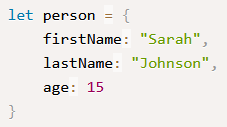Quiz - JavaScript Variables & Data Types
What are variables in JavaScript (and any other programming language)? *
10 pointsIf this is the blocks code for adding 1 to the 'score' variable, which of these is the JavaScript code to do the same thing? *
10 pointsIf this is the blocks code for showing the value of the 'score' variable, which of these is the JavaScript code to do the same thing? *
10 pointsWhat value will the 'fruit' variable be equal to after this code has run? *
10 points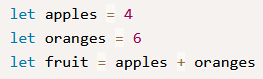What value will the 'fullname' variable be equal to after this code has run? *
10 points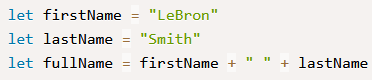The names that we give to variables are called identifiers and they have certain rules for how we can name them. Which one of these is a correct identifier? *
10 points
What data type is this variable? *
10 points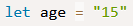How many possible values can a boolean variable have? *
10 points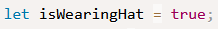What are arrays in JavaScript (and any other programming language)? *
10 points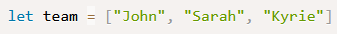The following code creates and sets up an object in the variable called 'person'. What are the properties in the object called? *
10 points